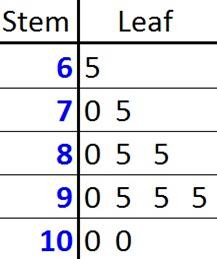© Ms. Garcia Math 7th The stem-and- leaf plot below shows test scores in Math of 12 students. GR34 answersUse the stem and leaf plot to answer these questions. 1) According to the plot, what is the maximum score? 2) According to the plot, what is the minimum score? 100 65 Answer Answer 3) According to the plot, how many students scored above 85? 4) According to the plot, how many students scored below 70? 6 1 Answer Answer Name: ID: